# Texas Go Math Grade 5 Lesson 13.2 Answer Key Customary Length

Refer to our Texas Go Math Grade 5 Answer Key Pdf to score good marks in the exams. Test yourself by practicing the problems from Texas Go Math Grade 5 Lesson 13.2 Answer Key Customary Length.

## Texas Go Math Grade 5 Lesson 13.2 Answer Key Customary Length

Essential Question
How can you compare and convert units of length?
To convert a smaller unit to a larger unit (eg to ), divide it by the number of smaller units that are needed to make. To convert from a larger unit to a smaller one, multiply. To convert from a smaller unit to a larger one, divide.

Unlock the Problem

To build a new swing, Mr. Mattson needs 9 feet of rope for each side of the swing and 6 more feet for the monkey bar. The hardware store sells rope by the yard.

A.
How many feet of rope does Mr. Mattson need for the swing?
It is given that
To build a new swing, Mr. Mattson needs 9 feet of rope for each side of the swing
Now,
We know that,
A swing has 2 sides
So,
The total length of the rope Mr. Mattson needs for the swing = 9 + 9 = 18 feet
Hence, from the above,
We can conclude that
The number of feet of rope does Mr. Mattson need for the swing is: 18 feet

B.
How many feet does Mr. Mattson need for the swing and the monkey bar combined?
It is given that
Mr. Mattson needs 9 feet of rope for each side of the swing and 6 more feet for the monkey bar
So,
The combined length of the rope Mr. Mattson needed = The total length of the rope needed for both ends of the swing + The length of the rope needed for the monkey bar
= 18 + 6
= 24 feet
Hence, from the above,
We can conclude that
The combined length of the rope Mr. Mattson needed is: 24 feet

Mr. Mattson needs to find how many yards of rope he needs to buy. He will need to convert 24 feet to yards. 1 low many groups of 3 feet are in 24 feet?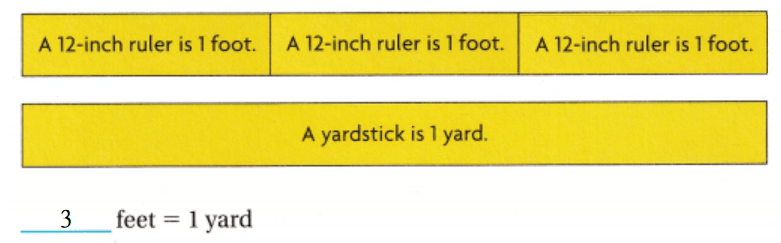Use a strip diagram to write an equation.So,
Mr. Mattson needs to buy 8 yards of rope.Math Talk
Mathematical Processes
What operation did you use when you found groups of 3 feet in 24 feet? Do you multiply or divide when you convert a smaller unit to a larger unit? Explain.
When you have to find the number of groups of 3 feet in 24 feet, the operation we will use is: Division
To convert a smaller unit to a larger unit (eg to ), divide it by the number of smaller units that are needed to make. To convert from a larger unit to a smaller one, multiply. To convert from a smaller unit to a larger one, divide.

Example

Use the table to find the relationship between miles and feet.

The distance between the new high school and the football field is 2 miles. How does this distance compare to 10,000 feet?
When you convert larger units to smaller units, you need to multiply.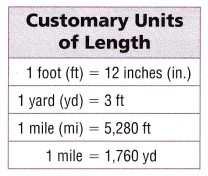Step 1
Convert 2 miles to feet.
Think: 1 mile is equal to 5,280 feet.
I need to multiply the total number of miles by 5,280 feetSo,
2 miles = 10,560 feet.

Step 2: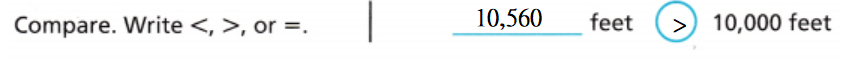Since 10,560 is greater than 10,000, the distance between the new high school and the football field is greater than 10,000 feet.

• Sometimes you need to convert a single unit of measurement to mixed measures. Convert 62 inches into feet and inches.
Think: 1 foot is equal to 12 inches.
62 ÷ 12 = 5 R 2 or 5 ft 2 in.

Share and Show

Convert.

Question 1.
2 mi = ___ yd
The given unit is: Miles
The conversion unit must be: Yards
Now,
From the given table,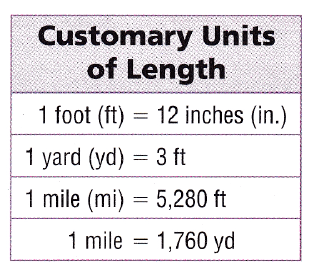We can observe that
1 mile = 1,760 yd
So,
2 miles = 2 × 1,760 yd
= 3,520 yd
Hence, from the above,
We can conclude that
2 miles = 3,520 yards

Question 2.
6 yd = __ ft.
The given unit is: Yards
The conversion unit must be: Feet
Now,
From the given table,We can observe that
1 yard = 3 feet
So,
6 yards = 6 × 3 feet
= 18 feet
Hence, from the above,
We can conclude that
6 yards = 18 feet

Question 3.
90 in. = ___ ft ___ in.
The given unit is: Inches
The conversion unit must be: Feet
Now,
From the given table,We can observe that
1 Inch = $$\frac{1}{12}$$ feet
So,
90 inches = 90 × $$\frac{1}{12}$$ feet
= 7 R 6
= 7 feet 6 inches
Hence, from the above,
We can conclude that
90 inches = 7 feet 6 inches

Math talk
Mathematical Processes
Explain how you know to measure it.

Practice: Copy and Solve Convert.

Question 4.
60 in. = __ ft
The given unit is: Inches
The conversion unit must be: Feet
Now,
From the given table,We can observe that
1 inch = $$\frac{1}{12}$$ feet
So,
60 inches = 60 × $$\frac{1}{12}$$ feet
= 5 feet
Hence, from the above,
We can conclude that
60 inches = 5 feet

Question 5.
__ ft = 7 yd
The given unit is: yard
The conversion unit must be: Feet
Now,
From the given table,We can observe that
1 Yard = 3 feet
So,
7 yards = 7 × 3 feet
= 21 feet
Hence, from the above,
We can conclude that
21 feet = 7 yards

Question 6.
4 mi = ___yd
The given unit is: Miles
The conversion unit must be: Yards
Now,
From the given table,We can observe that
1 mile = 1,760 yd
So,
4 miles = 4 × 1,760 yd
= 7,040 yd
Hence, from the above,
We can conclude that
4 miles = 7,040 yards

Question 7.
120 in. = __ ft
The given unit is: Inches
The conversion unit must be: Feet
Now,
From the given table,We can observe that
1 Inch = $$\frac{1}{12}$$ feet
So,
120 inches = 120 × $$\frac{1}{12}$$
= 10 feet
Hence, from the above,
We can conclude that
120 inches = 10 feet

Question 8.
46 ft = __ yd __ft
The given unit is: Feet
The conversion unit must be: Yards
Now,
From the given table,We can observe that
1feet = $$\frac{1}{3}$$ yd
So,
46 feet = 46 × $$\frac{1}{3}$$ yd
= 15 R 1
= 15 yards 1 feet
Hence, from the above,
We can conclude that
46 feet = 15 yards 1 feet

Question 9.
42 yd = __ ft
The given unit is: yards
The conversion unit must be: Feet
Now,
From the given table,We can observe that
1 Yard = 3 feet
So,
42 yards = 42 × 3 feet
= 126 feet
Hence, from the above,
We can conclude that
42 yards = 126 feet

Use Symbols Compare. Write <> or =.

Question 10.
8 ft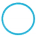3yd
The given units are: Feet, Yard
Now,
The given table is:Now,
From the given table,
We can observe that
3 yards = 3 × 3 feet
= 9 feet
So,
8 feet < 9 feet
Hence, from the above,
We can conclude that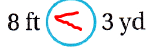Question 11.
2 mi10,500 ft
The given units are: Mile, Feet
Now,
The given table is:Now,
From the given table,
We can observe that
2 miles = 2 × 5,280 feet
= 10,560 feet
So,
10,560 feet > 10,500 feet
Hence, from the above,
We can conclude thatQuestion 12.
3 yd 2 ft132 in.
The given units are: Yard, Feet, and Inch
Now,
The given table is:Now,
From the given table,
We can observe that
3 yards 2 feet = (3 × 3 feet) + 2 feet
= 11 feet
= 11 × 12 inches
132 inches
So,
132 inches = 132 inches
Hence, from the above,
We can conclude thatQuestion 13.
H.O.T. Representations Make a table that shows the number of feet and inches in 1, 2, 3, and 4 yards. What do you notice about the relationship between the number of larger units and the number of smaller units as the length increases?
It is given that
Make a table that shows the number of feet and inches in 1, 2, 3, and 4 yards.
Now,
The representation of the given values of yards in terms of feet and inches is:Now,
From the above table,
We can observe that
As the length in yards inches,
The number of larger units increases as the multiples of 3
The number of smaller units increases as the multiples of 12
Hence, from the above,
We can conclude that
The number of larger units increases as the multiples of 3
The number of smaller units increases as the multiples of 12

Problem Solving.

Question 14.
H.O.T. Multi-Step Javon is helping his dad build a treehouse. He has a piece of trim that ¡s 13 feet long. How many pieces can Javon cut that is 1 yard long? How much of a yard will he have leftover?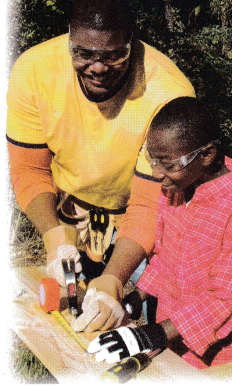It is given that
Javon is helping his dad build a treehouse. He has a piece of trim that ¡s 13 feet long
Now,
The given table is:Now,
From the given table,
We can observe that
1 feet = $$\frac{1}{3}$$ yards
So,
13 feet = 13 × $$\frac{1}{3}$$ yards
= 4 R 1
= 4 yards 1 foot
Hence, from the above,
We can conclude that
The number of pieces can Javon cut that is 1 yard long is: 4 pieces
The amount of a yard that Javon will have leftover is: 1 piece

Question 15.
H.O.T. Multi-Step Patty is building a rope ladder for a treehouse. She needs two 5-foot pieces of rope for the sides of the ladder. She needs 7 pieces of rope, each 18 inches long for the steps. How many feet of rope does Patty need to make the ladder? Write your answer as a mixed number and as a mixed measure in feet and inches.It is given that
Patty is building a rope ladder for a treehouse. She needs two 5-foot pieces of rope for the sides of the ladder. She needs 7 pieces of rope, each 18 inches long for the steps
Now,
We know that,
1 inch = $$\frac{1}{12}$$ feet
So,
The number of feet of rope does Patty need to make the ladder = (The number of feet of the rope needed for the sides of the ladder) + (The number of feet of rope needed for the steps of the ladder)
= (2 × 5 feet) + (7 × 18 inches)
= 10 feet + 126 inches
= 10 feet + 126 × $$\frac{1}{12}$$
= 10 feet + 10 R 6
= 10 feet + 10 feet 6 inches
= 20 R 6
= 20 feet 6 inches
Hence, from the above,
We can conclude that
The number of feet of rope does Patty need to make the ladder is: 20 feet 6 inches

Question 16.
Kelly lives 2 miles from school. Harry lives closer to the school than Kelly. Which could be the distance that Harry lives from school?
(A) 3,800 yards
(B) 10,560 feet
(C) 10,600 feet
(D) 3,461 yards
It is given that
Kelly lives 2 miles from school. Harry lives closer to the school than Kelly
Now,
The given table is:Now,
From the above table,
We can observe that
2 miles = 2 × 5,280 feet (or) 2 × 1,760 yd
= 10,560 feet (or) 3,520 yd
Hence, from the above,
We can conclude that
The distance that Harry lives from school is: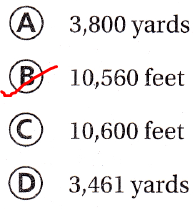Question 17.
Bart measured a piece of wood to be 9 feet long. How could he calculate the length of the wood in inches?
(A) 9 ÷ 12
(B) 9 × 12
(C) 12 + 9
(D) 12 ÷ 9
It is given that
Bart measured a piece of wood to be 9 feet long
Now,
We know that,
1 inch = $$\frac{1}{12}$$ feet
1 feet = 12 inches
So,
The length of the wood in inches = 9 × 12 inches
Hence, from the above,
We can conclude that
The length of the wood in inches is: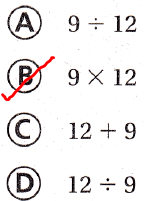Question 18.
Multi-Step Aliesha has a 55-inch rope. Mike has a rope that is 17 inches long. what is the length of Mike’s rope?
(A) 1 yard
(B) 5 feet
(C) 2 yards
(D) 7 feet
It is given that
Aliesha has a 55-inch rope. Mike has a rope that is 17 inches long
Now,
According to the given information,
The length of Mike’s rope = 55 + 17
= 72 inches
Now,
The given table is:Now,
From the given table,
We can observe that
72 inches = 72 ×$$\frac{1}{12}$$ feet
= 6 feet
6 feet = 6 × $$\frac{1}{3}$$ yd
= 2 yards
Hence, from the above,
We can conclude that
The length of Mike’s rope is: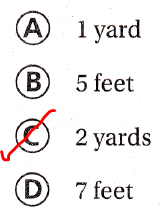Texas Test Prep

Question 19.
Katy’s driveway is 120 feet long. How many yards long is Katy’s driveway?
(A) 60 yards
(B) 40 yards
(C) 20 yards
(D) 10 yards
It is given that
Katy’s driveway is 120 feet long
Now,
We know that,
1 feet = $$\frac{1}{3}$$ yards
So,
The length of Kathy’s driveway in yards = 120 × $$\frac{1}{3}$$ yards
= 40 yards
Hence, from the above,
We can conclude that
The length of Kathy’s driveway in yards is:### Texas Go Math Grade 5 Lesson 13.2 Homework and Practice Answer Key

Convert.

Question 1.
3 mi = __ yd
The given unit is: Miles
The conversion unit must be: Yards
Now,
From the given table,We can observe that
1 Mile = 1,760 Yards
So,
3 miles = 3 × 1,760 yards
= 5,280 yards
Hence, from the above,
We can conclude that
3 miles = 5,280 yards

Question 2.
72 in. = ___ ft
The given unit is: Inches
The conversion unit must be: Feet
Now,
From the given table,We can observe that
1 Inch = $$\frac{1}{12}$$ feet
So,
72 inches = 72 × $$\frac{1}{12}$$ feet
= 6 feet
Hence, from the above,
We can conclude that
72 inches = 6 feet

Question 3.
__ ft = 8 yd
The given unit is: yards
The conversion unit must be: Feet
Now,
From the given table,We can observe that
1 Yard = 3 feet
So,
8 yards = 8 × 3 feet
= 24 feet
Hence, from the above,
We can conclude that
24 feet = 8 yards

Question 4.
132 in. = __ ft
The given unit is: Inches
The conversion unit must be: Feet
Now,
From the given table,We can observe that
1 Inch = $$\frac{1}{12}$$ feet
So,
132 inches = 132 × $$\frac{1}{12}$$ feet
= 11 feet
Hence, from the above,
We can conclude that
132 inches = 11 feet

Question 5.
36 ft = __ yd
The given unit is: Feet
The conversion unit must be: Yards
Now,
From the given table,We can observe that
1 Feet = $$\frac{1}{3}$$ yards
So,
36 feet = 36 × $$\frac{1}{3}$$ yards
= 12 yards
Hence, from the above,
We can conclude that
36 feet = 12 yards

Question 6.
46 yd = __ ft
The given unit is: yards
The conversion unit must be: Feet
Now,
From the given table,We can observe that
1 Yard = 3 feet
So,
46 yards = 46 × 3 feet
= 138 feet
Hence, from the above,
We can conclude that
46 yards = 138 feet

Question 7.
15 ft = __ in.
The given unit is: Feet
The conversion unit must be: Inches
Now,
From the given table,We can observe that
1 Feet = 12 inches
So,
15 feet = 15 × 12 inches
= 180 inches
Hence, from the above,
We can conclude that
15 feet = 180 inches

Question 8.
__ ft = 24 yd 2 ft
The given unit is: yards
The conversion unit must be: Feet
Now,
From the given table,We can observe that
1 Yard = 3 feet
So,
24 yards 2 feet = (24 × 3 feet) + 2 feet
= 74 feet
Hence, from the above,
We can conclude that
74 feet = 24 yards 2 feet

Question 9.
__ ft = 7 mi
The given unit is: Miles
The conversion unit must be: Feet
Now,
From the given table,We can observe that
1 Mile = 5,280 feet
So,
7 miles = 7 × 5,280 feet
= 36,960 feet
Hence, from the above,
We can conclude that
36,960 feet = 7 miles

Compare. Write <, >, or =.

Question 10.
2 mi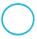3,526 yd
The given units are: Mile, Yard
Now,
The given table is:Now,
From the given table,
We can observe that
2 miles = 2 × 1,760 yards
= 3,520 yards
So,
3,520 yards < 3,526 yards
Hence, from the above,
We can conclude thatQuestion 11.
4 yd13 ft
The given units are: Yard, Feet
Now,
The given table is:Now,
From the given table,
We can observe that
4 yards = 4 × 3 feet
= 12 feet
So,
12 feet < 13 feet
Hence, from the above,
We can conclude that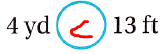Question 12.
5 mi26,300 ft
The given units are: Mile, Feet
Now,
The given table is:Now,
From the given table,
We can observe that
5 miles = 5 × 5,280 feet
= 26,400 feet
So,
26,400 feet > 26,300 feet
Hence, from the above,
We can conclude thatQuestion 13.
90 ft32 yd
The given units are: Feet, Yard
Now,
The given table is:Now,
From the given table,
We can observe that
32 yards = 32 × 3 feet
= 96 feet
So,
90 feet < 96 feet
Hence, from the above,
We can conclude thatQuestion 14.
27 ft324 in.
The given units are: Feet, Inch
Now,
The given table is:Now,
From the given table,
We can observe that
27 feet = 27 × 12 inches
= 324 inches
So,
324 inches = 324 inches
Hence, from the above,
We can conclude that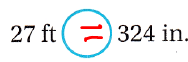Question 15.
5 yd 2 ft200 in.
The given units are: yard, Feet, and Inch
Now,
The given table is:Now,
From the given table,
We can observe that
5 yard 2 feet = (5 × 3 feet) + 2 feet
= 17 feet
17 feet = 17 × 12 inches
= 204 inches
So,
204 inches > 200 inches
Hence, from the above,
We can conclude thatProblem Solving

Question 16.
A prickly pear cactus is 1 yard tall. A yucca plant is 35 inches tall. Which plant is taller? Tell me how you know.
It is given that
A prickly pear cactus is 1 yard tall. A yucca plant is 35 inches tall
Now,
The given table is:Now,
From the given table,
We can observe that
1 yard = 3 feet
1 feet = 12 inches
So,
3 feet = 3 ×12 inches
= 36 inches
Now,
Since both the values of the heights have the same unit measures, compare both the heights
So,
36 inches > 35 inches
Hence, from the above,
We can conclude that
A prickly pear cactus is taller than a Yucca plant

Question 17.
The length of the Beecher family’s driveway is 20 yd. The length of their SUV is one-fourth the length of the driveway. How many feet long is the SUV?
It is given that
The length of the Beecher family’s driveway is 20 yd. The length of their SUV is one-fourth the length of the driveway
Now,
We know that,
1 yard = 3 feet
So,
20 yards = 20 × 3 feet
= 60 feet
So,
The length of the Beecher’s family driveway is: 60 feet
Now,
According to the given information,
The length of their SUV = $$\frac{60 feet}{4}$$
= 15 feet
Hence, from the above,
We can conclude that
The length of the Beecher’s family’s SUV is: 15 feet

Lesson Check

Texas Test Prep

Question 18.
The length of a cruise ship is 1,092 feet. How many yards long is the cruise ship?
(A) 3,276 yards
(B) 91 yards
(C) 330 yards
(D) 364 yards
It is given that
The length of a cruise ship is 1,092 feet
Now,
The given table is:Now,
From the above table,
We can observe that
1 feet = $$\frac{1}{3}$$ yards
So,
1,092 feet = 1,092 × $$\frac{1}{3}$$
= 364 yards
Hence, from the above,
We can conclude that
The length of the cruise ship in terms of yards is:Question 19.
Nadia runs 8 miles each weekend. How many yards does Nadia run each weekend?
(A) 14,080 yards
(B) 42,240 yards
(C) 14,960 yards
(D) 220 yards
It is given that
Nadia runs 8 miles each weekend
Now,
The given table is:Now,
From the given table,
We can observe that
1 mile = 1,760 yards
So,
8 miles = 8 × 1,760 yards
= 14,080 yards
Hence, from the above,
We can conclude that
The number of yards does Nadia run each weekend is: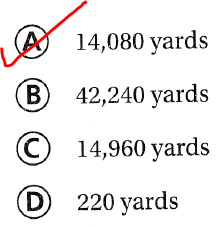Question 20.
Since the 1980 eruption of Mount St. Helens in Washington state, the elevation of its summit is about 1,300 feet lower. How can you calculate the difference ¡n elevation in inches?
(A) 1,300 × 12
(B) 1,300 ÷ 12
(C) 1,300 × 3
(D) 1,300 – 12
It is given that
Since the 1980 eruption of Mount St. Helens in Washington state, the elevation of its summit is about 1,300 feet lower
Now,
We know that,
1 feet = 12 inches
So,
The elevation of the summit in inches = 1,300 × 12
Hence, from the above,
We can conclude that
The elevation of the summit in inches is:Question 21.
The height of the U.S. Capitol building from the ground to the top of the Statue of Freedom is 96 yards. The height of the Texas State Capitol building is greater than the U.S. Capitol. Which could be the height of the Texas State Capitol?
(A) 310 feet
(B) 288 feet
(C) 3,452 inches
(D) 3,420 inches
It is given that
The height of the U.S. Capitol building from the ground to the top of the Statue of Freedom is 96 yards. The height of the Texas State Capitol building is greater than the U.S. Capitol
Now,
We know that,
1 yard = 3 feet
1 feet = 12 inches
So,
The height of the U.S. Capitol building = 96 × 3 feet
= 288 feet
= 288 × 12
= 3,456 inches
Hence, from the above,
We can conclude that
The height of the Texas State Capitol building is: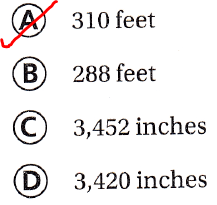Question 22.
Multi-Step Tyler’s older brother Joseph is 5 feet 6 inches tall. Joseph is 16 inches taller than Tyler. How tall is Tyler?
(A) 4 ft 2 in.
(B) 4 ft
(C) 6 ft 10 in.
(D) 6 ft 4 in.
It is given that
Tyler’s older brother Joseph is 5 feet 6 inches tall. Joseph is 16 inches taller than Tyler
Now,
We know that,
1 feet = 12 inches
So,
The height of Joseph = 5 feet 6 inches
= (5 × 12) + 6
= 60 + 6
= 66 inches
Now,
According to the given information,
The height of Tyler = 66 – 16
= 50 inches
= 4 feet 2 inches
Hence, from the above,
We can conclude that
The height of Tyler is: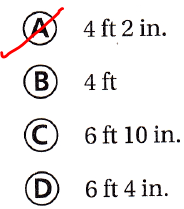Question 23.
Multi-Step Calvin fishes from a dock that is 85 inches long. Which of the following is equal to 85 inches?
(A) 2 yd 12 ft 1 in.
(B) 2 yd 1 ft 1 in.
(C) 1 yd 3 ft 1 in.
(D) 1 yd 4 ft 3 in.
It is given that
Calvin fishes from a dock that is 85 inches long.
Now,
The given table is:Now,
We know that,
1 inch = $$\frac{1}{12}$$ feet
1 yard = 3 feet
So,
85 inches = 85 × $$\frac{1}{12}$$
= 7 R 1
= 7 feet 1 inch
= 2 yards 1 feet 1 inch
Hence, from the above,
We can conclude that
The measurement that is equal to 85 inches is: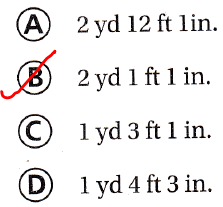Scroll to Top